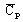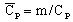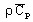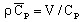# Heat capacity: definitions

## Definition

The heat capacity of a material is the heat quantity necessary to raise its temperature by one degree.

The mathematical relationship expressing this definition has the form:

ΔQ = Cp . ΔT

where:

• ΔQ is the heat quantity (J)
• ΔT is the temperature variation (in degrees)
• Cp is the heat capacity at constant pressure (J/degree)

The heat capacity of a body is a quantity that offers the possibility to evaluate the amount of heat exchanged by this body during a process characterized by a temperature variation.

## Specifications

The heat capacity Cp is an extensive quantity.

Thus in practice, the following quantities will be used:

• specific heat capacity1:with(J/kg/degree)

• volumetric heat capacity2:with(J/m3/degree)

Note: 1 Also called specific heat
Note: 2 In Flux the volumetric heat capacity has the notation ρCp

## Dependence on temperature

The heat capacity can depend on the temperature: Cp=f(T). It increases as the temperature increase.

The variation of the heat capacities with temperature can provide important information about the crystalline microstructure of the material (see § Phase transition ).